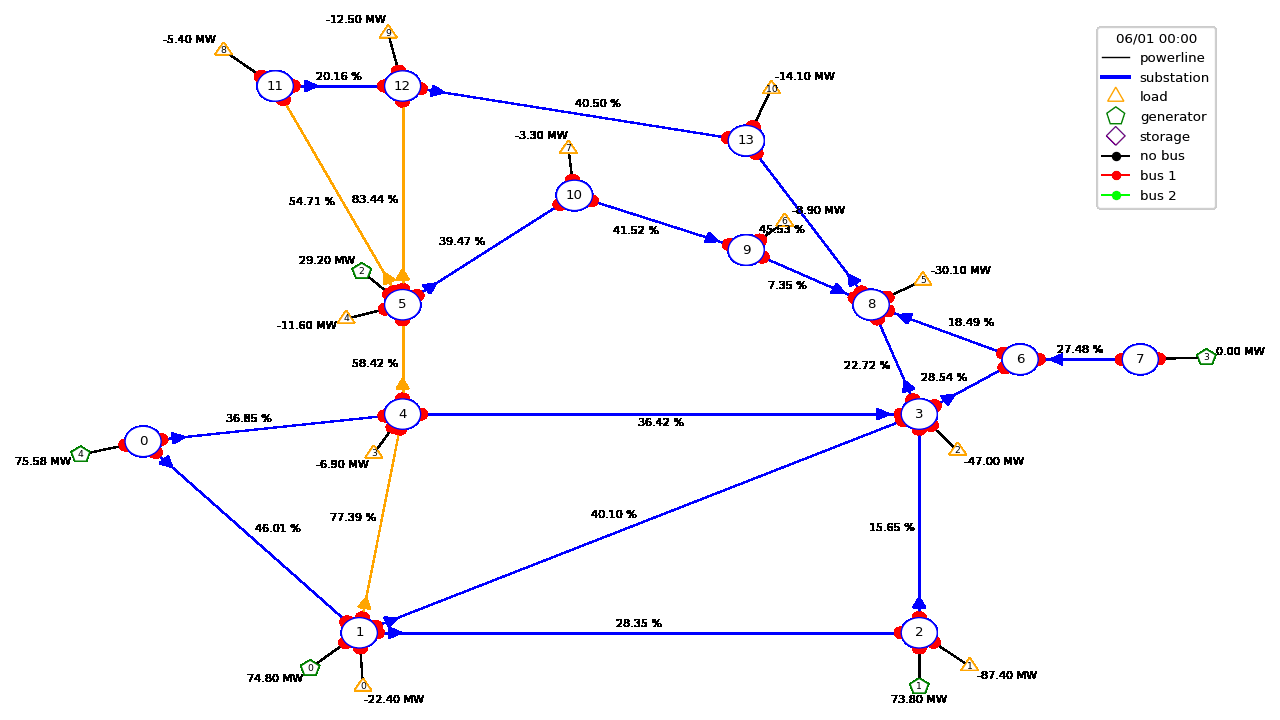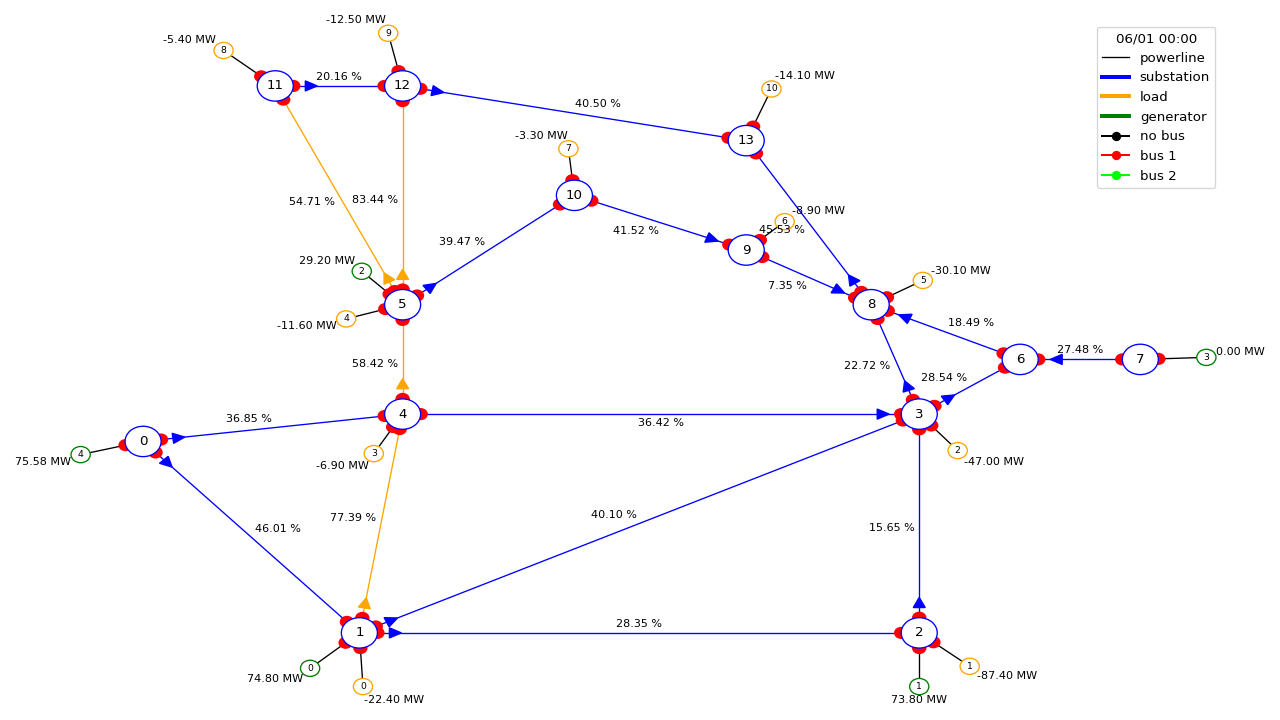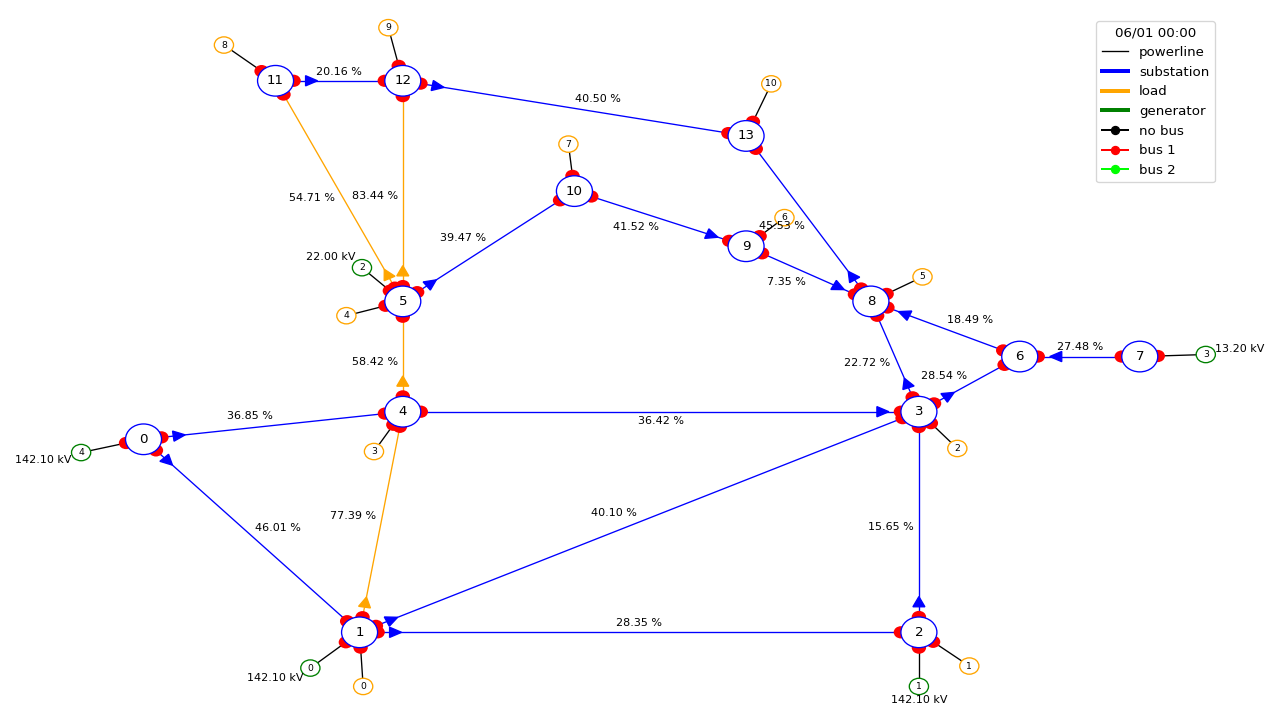# Grid2Op Plotting capabilities (beta)¶

## Objectives¶

This module contrains all the plotting utilities of grid2op. These utilities can be used in different manners to serve different purposes:

• `PlotMatplot` allows a more in depth study, with a better overall layout. It uses the well-known matplotlib library to represent the powergrid on the screen.

• `PlotPlotly` uses plotly library to represent the graph. As opposed to the others, plotly allows dynamic modifications such as zoom in / out. This makes this class particularly suited for in depth study of some powergrid state.

The class `PlotMatplot` is also used by `EpisodeReplay` that allows to look at the action taken by the agent pretty easily, and allows easy saving into gif format (see below for more information)

```import os
import warnings
import grid2op
from grid2op.Episode import EpisodeReplay
from grid2op.Agent import GreedyAgent, RandomAgent
from grid2op.Runner import Runner
from tqdm import tqdm

path_agents = "agent_pseudo_random"
with warnings.catch_warnings():
warnings.filterwarnings("ignore")
env = grid2op.make("rte_case14_realistic")

class CustomRandom(RandomAgent):
"""
This agent takes 1 random action every 10 time steps.
"""
def __init__(self, action_space):
RandomAgent.__init__(self, action_space)
self.i = 0

def my_act(self, transformed_observation, reward, done=False):
if self.i % 10 != 0:
res = 0
else:
res = self.action_space.sample()
self.i += 1
return res

# execute this agent on 1 scenario, saving the results
runner = Runner(**env.get_params_for_runner(), agentClass=CustomRandom)
path_agent = os.path.join(path_agents, "RandomAgent")
res = runner.run(nb_episode=1, path_save=path_agent, pbar=tqdm, agent_seeds=)
# and now reload it and display the "movie" of this scenario
plot_epi = EpisodeReplay(path_agent)
plot_epi.replay_episode(res, gif_name="episode")
```

An possible output will look like this:## Render the state of the grid¶

### During the gym loop¶

In Grid2Op we also made available the possibility to render the state of the grid that your agent sees before taking an action. This can be done with the provided environments following openAI gym interface like this:

```import grid2op
from grid2op.Agent import RandomAgent
from grid2op.Episode import EpisodeReplay

env = grid2op.make()
agent = RandomAgent(env.action_space)
nb_episode = 1
for i in range(nb_episode):
obs = env.reset()
done = False
reward = env.reward_range
while not done:
_ = env.render()
act = agent.act(obs, reward, done)
obs, reward, done, info = env.step(act)
```

NB we don’t recommend to use the renderer during the training of an Agent as it might slow down the training significantly.

### Offline, after the scenarios were played¶

In Grid2Op, you can execute a Runner to perform the “gym loops” and store the results in a standardized manner. Once stored, the results can be loaded back and “replayed” using the appropriate class. Here is how you can do this:

```import grid2op
from grid2op.Agent import RandomAgent
from grid2op.Runner import Runner
from grid2op.Episode import EpisodeReplay

path_saved_data = "where_i_want_to_save_it"
# create an environment and an agent
env = grid2op.make()
agent = RandomAgent(env.action_space)

# create a runner
runner = Runner(**env.get_params_for_runner(), agentClass=None, agentInstance=agent)

# run and save the results
res = runner.run(nb_episode=1, path_save=path_saved_data)

# and now load it and play the "movie"
plot_epi = EpisodeReplay(path_saved_data)
plot_epi.replay_episode(res, gif_name="this_episode.gif")

# and in `os.path.join(path_saved_data, res)` (or example "where_i_want_to_save_it/000")
# a file named "this_episode.gif" has been created
```

### Plot a given observation¶

We also included some module to plot a given observation that can be customize depending on what you want to plot.

You can use them as follow:

```import grid2op
from grid2op.PlotGrid import PlotMatplot

env = grid2op.make()
plot_helper = PlotMatplot(env.observation_space)

obs = env.reset()

# if you want to plot all the observation
fig = plot_helper.plot_obs(obs)
fig.show()

# you can also chose what to plot for each "object type"
fig_custom = plot_helper.plot_obs(obs
line_info="rho",
gen_info="v"  # i draw the voltage setpoint of the generators
)
fig_custom.show()
```

See definition of `BasePlot.plot_obs()` for more information. The results of the above code is:And the second image is### Plot data on the grid¶

For convenience, we also included a set of function that are able to plot some custom information and “project” them into the graph of the grid. This can be interesting if you want to represent some properties of the objects on the grid. The function showed here accept anything that can be converted to `float` that have the same size of the number of objects (for example if you want to display something on the powerlines you need this “something” to be vector that counts as many elements as the number of powerlines in the powergrid).

In the example bellow, we plot the thermal limits (maximum current allowed on a powerline) of the each powerline:

```import grid2op
from grid2op.PlotGrid import PlotMatplot

env = grid2op.make()
plot_helper = PlotMatplot(env.observation_space)

# plot the thermal limits of each powerlines
fig_info = plot_helper.plot_info(line_values=env.get_thermal_limit())
fig_info.show()
```

The above code will output this imageOf course you can also “project” on the grid all kind of variable and also for generators and loads, for example with

```import grid2op
from grid2op.PlotGrid import PlotMatplot

env = grid2op.make()
plot_helper = PlotMatplot(env.observation_space)

# plot the thermal limits of each powerlines and the voltages magnitude of each load
fig_info.show()

# plot only the generator pmax
fig_info2 = plot_helper.plot_info(gen_values=env.gen_pmax)
fig_info2.show()
```

More information is available in the description of the function `BasePlot.plot_info()`.

## Detailed Documentation by class¶

Classes:

 `BasePlot`(observation_space[, width, height, ...]) INTERNAL
class grid2op.PlotGrid.BasePlot(observation_space, width=800, height=600, scale=2000.0, grid_layout=None, parallel_spacing=3.0)[source]

INTERNAL

Warning

/!\ Internal, do not use unless you know what you are doing /!\

Abstract interface to plot the state of the powergrid

Implement the interface with a plotting library to generate drawings

observation_space

The observation space used.

Type:

`grid2op.Observation.ObservationSpace`

width
Type:

`int` Width of the drawing

height
Type:

`int` Height of the drawing

grid_layout
Type:

`dict` A grid layout dict to use

Methods:

 `__init__`(observation_space[, width, height, ...]) `_aux_draw_elements`(figure, observation, ...) generic method to loop through all elements of a given type and call the draw function on them `clear_figure`(figure) Clears a figure Depending on the library can also be called Plot, canvas, screen .. `compute_grid_layout`(observation_space[, ...]) Compute the grid layout from the observation space `convert_figure_to_numpy_HWC`(figure) Given a figure as returned by BasePlot.create_figure Convert it to a numpy array of dtype uint8 and data layed out in the HWC format `create_figure`() Creates a new figure to draw into. `draw_gen`(figure, observation, gen_name, ...) Draws a generator into the figure `draw_legend`(figure, observation) Setup the legend for the given figure. `draw_load`(figure, observation, load_name, ...) Draws a load into the figure `draw_powerline`(figure, observation, line_id, ...) Draws a powerline into the figure `draw_storage`(figure, observation, ...) Draws a storage unit into the figure `draw_substation`(figure, observation, sub_id, ...) Draws a substation into the figure `plot_info`([figure, redraw, line_values, ...]) Plot an observation with custom values `plot_layout`() This function plot the layout of the grid, as well as the object. `plot_obs`(observation[, figure, redraw, ...]) Plot an observation. `plot_postprocess`(figure, observation, is_update) Some implementations may need post-processing. `update_gen`(figure, observation, gen_name, ...) INTERNAL `update_legend`(figure, observation) INTERNAL `update_load`(figure, observation, load_name, ...) INTERNAL `update_powerline`(figure, observation, ...) INTERNAL `update_storage`(figure, observation, ...) INTERNAL `update_substation`(figure, observation, ...) INTERNAL

Attributes:

 `__weakref__` list of weak references to the object (if defined)
__init__(observation_space, width=800, height=600, scale=2000.0, grid_layout=None, parallel_spacing=3.0)[source]
__weakref__

list of weak references to the object (if defined)

generic method to loop through all elements of a given type and call the draw function on them

abstractmethod clear_figure(figure)[source]

Clears a figure Depending on the library can also be called Plot, canvas, screen ..

compute_grid_layout(observation_space, grid_layout=None)[source]

Compute the grid layout from the observation space

This should return a native python `dict` in the same format as observation_space.grid_layout :

```{
"substation1_name": [x_coord, y_coord],
"substation2_name": [x_coord, y_coord],
[...],
[...],
"gen1_name": [x_coord, y_coord],
[...]
}
```

Note that is must contain at least the positions for the substations. The loads and generators will be skipped if missing.

By default, if grid_layout is provided this is returned, otherwise returns observation_space.grid_layout

Parameters:
• observation_space (`grid2op.Observation.ObservationSpace`) – The observation space of the environment

• grid_layout (`dict` or `None`) – A dictionary containing the coordinates for each substation.

abstractmethod convert_figure_to_numpy_HWC(figure)[source]

Given a figure as returned by BasePlot.create_figure Convert it to a numpy array of dtype uint8 and data layed out in the HWC format

abstractmethod create_figure()[source]

Creates a new figure to draw into. Depending on the library can also be called Plot, canvas, screen ..

abstractmethod draw_gen(figure, observation, gen_name, gen_id, gen_bus, gen_value, gen_unit, pos_x, pos_y, sub_x, sub_y)[source]

Draws a generator into the figure

Parameters:
• figure

• create_figure (This is the object returned by) –

• observation (grid2op.Observation.BaseObservation) –

• drawn (Current state of the grid being) –

• gen_name (`str` Name of the load) –

• gen_id (`int` Id of the generator, Index in the observation) –

• gen_bus (`int` Bus id the generator is connected to) –

• gen_value (`float`) –

• state (An informative value of the generator current) –

• gen_unit (`str` The unit of the gen_value argument as a string) –

• pos_x (`int` x position from the layout) –

• pos_y (`int` y position from the layout) –

• sub_x (`int` x position of the connected substation from the layout) –

• sub_y (`int` y position of the connected substation from the layout) –

abstractmethod draw_legend(figure, observation)[source]

Setup the legend for the given figure.

Parameters:
• figure (`object` Figure to draw to.) –

• create_figure (This is the object returned by) –

• observation (`grid2op.Observation.BaseObservation`) –

• drawn (Current state of the grid being) –

Draws a load into the figure

Parameters:
• figure

• create_figure (This is the object returned by) –

• observation

• drawn (Current state of the grid being) –

• load_name (`str` Name of the load) –

• load_id (`int` Id of the load, Index in the observation) –

• load_bus (`int` Id of bus the load is connected to.) –

• load_value (`float` An informative value of the load current state) –

• load_unit (`str` The unit of the load_value argument as a string) –

• pos_x (`int` x position from the layout) –

• pos_y (`int` y position from the layout) –

• sub_x (`int` x position of the connected substation from the layout) –

• sub_y (`int` y position of the connected substation from the layout) –

abstractmethod draw_powerline(figure, observation, line_id, line_name, connected, line_value, line_unit, or_bus, pos_or_x, pos_or_y, ex_bus, pos_ex_x, pos_ex_y)[source]

Draws a powerline into the figure

Parameters:
• figure (`object` Figure to draw to.) –

• create_figure (This is the object returned by) –

• observation (`grid2op.Observation.BaseObservation`) –

• drawn (Current state of the grid being) –

• line_id (`int` Id of the powerline, index in the observation) –

• line_name (`str` Name of the powerline) –

• connected (`bool` Is the line connected ?) –

• line_value (`float` An informative value of the line current state) –

• line_unit (`str` The unit of the line_value argument as a string) –

• or_bus (`int` Bus the powerline origin is connected to) –

• pos_or_x (`int` Powerline origin x position from the layout) –

• pos_or_y (`int` Powerline origin y position from the layout) –

• ex_bus (`int` Bus the powerline extremity is connected to) –

• pos_ex_x (`int` Powerline extremity x position from the layout) –

• pos_ex_y (`int` Powerline extremity y position from the layout) –

abstractmethod draw_storage(figure, observation, storage_name, storage_id, storage_bus, storage_value, storage_unit, pos_x, pos_y, sub_x, sub_y)[source]

Draws a storage unit into the figure

Parameters:
• figure

• create_figure (This is the object returned by) –

• observation

• drawn (Current state of the grid being) –

• storage_name (`str` Name of the load) –

• storage_id (`int` Id of the load, Index in the observation) –

• storage_bus (`int` Id of bus the load is connected to.) –

• storage_value (`float` An informative value of the load current state) –

• storage_unit (`str` The unit of the load_value argument as a string) –

• pos_x (`int` x position from the layout) –

• pos_y (`int` y position from the layout) –

• sub_x (`int` x position of the connected substation from the layout) –

• sub_y (`int` y position of the connected substation from the layout) –

abstractmethod draw_substation(figure, observation, sub_id, sub_name, pos_x, pos_y)[source]

Draws a substation into the figure

Parameters:
• figure

• create_figure (This is the object returned by) –

• observation

• drawn (Current state of the grid being) –

• sub_id

• sub_name

• pos_x

• pos_y

plot_info(figure=None, redraw=True, line_values=None, line_unit='', load_values=None, load_unit='', storage_values=None, storage_unit='', gen_values=None, gen_unit='', observation=None, coloring=None)[source]

Plot an observation with custom values

Parameters:
• figure (`object`) – The figure on which to plot the observation. If figure is `None` a new figure is created.

• line_values (`list`) – information to be displayed for the powerlines [must have the same size as observation.n_line and convertible to float]

• line_unit (`str`) – Unit string for the :line_values: argument, displayed after the line value

• load_values (`list`) – information to display for the loads [must have the same size as observation.n_load and convertible to float]

• load_unit (`str`) – Unit string for the :load_values: argument, displayed after the load value

• storage_values (`list`) – information to display for the storage units [must have the same size as observation.n_storage and convertible to float]

• storage_unit (`str`) – Unit string for the :storage_values: argument, displayed after the storage value

• gen_values (`list`) – information to display in the generators [must have the same size as observation.n_gen and convertible to float]

• gen_unit (`str`) – Unit string for the :gen_values: argument, displayed after the generator value

• observation (`grid2op.Observation.BaseObservation`) – An observation to plot, can be None if no values are drawn from the observation

• coloring`None` for no special coloring, or “line” to color the powerline based on the value (“gen” and “load” coming soon)

Examples

More examples on how to use this function is given in the “8_PlottingCapabilities.ipynb” notebook.

The basic concept is:

```import grid2op
from grid2op.PlotGrid import PlotMatplot
env = grid2op.make()
plot_helper = PlotMatplot(env.observation_space)

# plot the layout (position of each elements) of the powergrid
plot_helper.plot_layout()

# project some data on the grid
line_values = env.get_thermal_limit()
plot_helper.plot_info(line_values=line_values)

# to plot an observation
obs = env.reset()
plot_helper.plot_obs(obs)
```
Returns:

res – The figure updated with the data from the new observation.

Return type:

`object`

plot_layout()[source]

This function plot the layout of the grid, as well as the object. You will see the name of each elements and their id.

plot_obs(observation, figure=None, redraw=True, line_info='rho', load_info='p', gen_info='p', storage_info='p')[source]

Plot an observation.

Parameters:
• observation (`grid2op.Observation.BaseObservation`) – The observation to plot

• figure (`object`) – The figure on which to plot the observation. If figure is `None`, a new figure is created.

• line_info (`str`) – One of “rho”, “a”, or “p” or “v” The information that will be plotted on the powerline. By default “rho”. All flow are taken “origin” side.

• load_info (`str`) – One of “p” or “v” the information displayed on the load. (default to “p”).

• gen_info (`str`) – One of “p” or “v” the information displayed on the generators (default to “p”).

• storage_info (`str`) – One of “p” or None the information displayed on the generators (default to “p”).

Returns:

res – The figure updated with the data from the new observation.

Return type:

`object`

plot_postprocess(figure, observation, is_update)[source]

Some implementations may need post-processing. This is called at the end of plot.

update_gen(figure, observation, gen_name, gen_id, gen_bus, gen_value, gen_unit, pos_x, pos_y, sub_x, sub_y)[source]

INTERNAL

Warning

/!\ Internal, do not use unless you know what you are doing /!\

Updates a generator into the figure

update_legend(figure, observation)[source]

INTERNAL

Warning

/!\ Internal, do not use unless you know what you are doing /!\

Updates the legend for the given figure.

INTERNAL

Warning

/!\ Internal, do not use unless you know what you are doing /!\

Update a load into the figure

update_powerline(figure, observation, line_id, line_name, connected, line_value, line_unit, or_bus, pos_or_x, pos_or_y, ex_bus, pos_ex_x, pos_ex_y)[source]

INTERNAL

Warning

/!\ Internal, do not use unless you know what you are doing /!\

Draws a powerline into the figure

update_storage(figure, observation, storage_name, storage_id, storage_bus, storage_value, storage_unit, pos_x, pos_y, sub_x, sub_y)[source]

INTERNAL

Warning

/!\ Internal, do not use unless you know what you are doing /!\

Update a storage unit into the figure

update_substation(figure, observation, sub_id, sub_name, pos_x, pos_y)[source]

INTERNAL

Warning

/!\ Internal, do not use unless you know what you are doing /!\

Update a substation into the figure

If you still can’t find what you’re looking for, try in one of the following pages:

Still trouble finding the information ? Do not hesitate to send a github issue about the documentation at this link: Documentation issue template